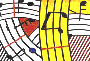Tutorial 24 - Voice I

Introduction to Voice

Topics

Voice rhythmical structure

Functions used

Voice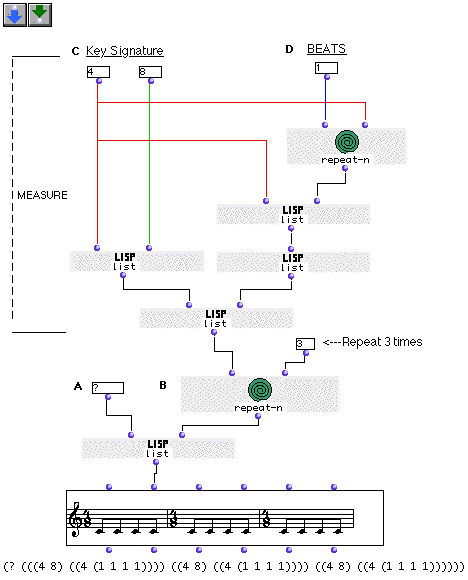Description

The VOICE object is a factory that deals with symbolic representation of rhythm. Here rhythm will be represented following a tree syntax (a list of lists). In this tutorial we will construct tree structures repesenting rhythmical notation. (For further information about rhytm trees c.f Appendix of the OpenMusic reference) .

The rhythmical tree structure

The second input of VOICE takes a list which will be represented in its editor as a notated rhythmical line. Now let us examine closely the special structure of this list:

This list is in fact a list of lists which is called a tree. This tree has a specific syntax:

• The first element of the list is a question mark '?', which stands for the given number of measures. Usually when a rhythmical tree is given by the user the question mark is a practical way of saying "this is a rhythm list with n measures' meaning undefined number of measure. (After evaluation, the VOICE object computes by itself the number of measures given and returns the correct integer which will replace the question mark sign).
• The second element of the list is the rhythmic structure representing a list of measures.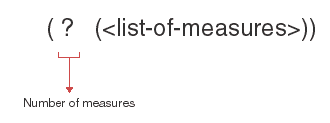• Each measure-list also contains two list: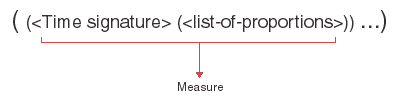• The first list is the time signature which can be represented in two different ways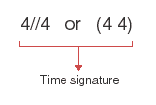• As a list (4 4) (meaning a measure of four quarter notes)
• Or in this way 4//4 (the same as above)

• The second list is a list of proportions. These will determine the rythmical structure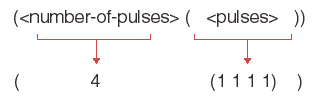The structure is again a list of lists where the first element is the number of pulses contained in the measure followed by its subdivision. (Here in the example above, we have four equal pulses ). We could have written (4 (3 1)) which represents in a 4//4 measure a dotted half note followed by a quarter note.

Rests

We might also represent in this level rests by using negative integers. Example:

(? ( ((4 8) ((4 ( 1 -2 1)))) ) ) will output :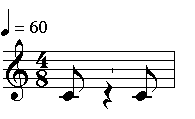Ties

In order to represent ties, one will use floats as follows:

(? ( ((4 8) ((4 ( 1 -2 1)))) ((3 4) ((3 (1.0 2)))) ) )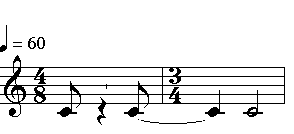Irrationals (Tuplets)

Irrationals such as tuplets, quintiplets etc.. are naturally represented by subdividing a pulse with a non-multiple . For instance if we subdivide one pulse into five beats ( instead of four) we will obtain a quintuplet:

Four beats resulting in four sixteeth notes:

(?(((1 4) ((1 (1 1 1 1)))) ))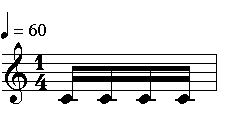Five beats giving a quinuplet of sixtenth notes:

(?(((1 4) ((1 (1 1 1 1 1)))) ))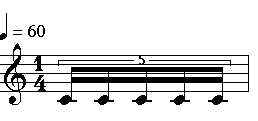Patch structure

In this tutorial's patch we will generate a simple rhythmical line representing regular eight notes pulses in three measures of 4 8. This will be done by using mainly the list function

A: In (A) we just add the question mark as the first element of the list.

B: in (B) we will repeat using repeat-n three times the list structure which represents measures.

C: A measure is again constructed using embeded lists in the correct order.